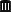## FDA guideline on BE, draft August 2021 [BE/BA News]

Hi ElMaestro,

THX, had a quick look.

» Draft.What else…Main difference is that reference-scaling is detailed and not just mentioning the progesterone guidance in a footnote – like in thedraft of 2013. Interesting difference:

Progesterone guidance

$$\small{\text{a. the 95% upper confidence bound for }\left(\widehat{Y}_T-\widehat{Y}_R\right)^2-\theta s_{wR}^2\text{ must be}\leq 0}$$

New ANDA draft

$$\small{\text{a. The 95% upper confidence bound for }\left(\widehat{Y}_T-\widehat{Y}_R\right)^2-\theta s_{wR}^2\text{ must be}\leq 0}$$
$$\small{\;\;\;\;\text{(numbers should be kept to a minimum of four significant figures for comparison).}}$$

(my emphasis)
We had a few discussions about rounding in RSABE in the past (here and there).

In both guidances the formula is confusing. The regulatory limit $$\small{\sigma_\textrm{w0}=0.25}$$ leads to the regulatory constant $$\small{\theta_\textrm{s}=\frac{\log_{e}1.25}{\sigma_\textrm{w0}}=0.89257\ldots}$$Furthermore, we need the standard error $$\small{s_\textrm{d}}$$ of the point estimate $$\small{PE=\widehat{Y}_\textrm{T}-\widehat{Y}_\textrm{R}}$$ given by $$\small{s_\textrm{d}=\sqrt{\frac{MSE}{seq^2}\sum{\frac{1}{n_i}}},}$$ where $$\small{MSE}$$ is the residual Mean Squares Error, $$\small{seq}$$ the number of sequences, and $$\small{n_i}$$ are the number of subjects in sequence $$\small{i}$$. According to the SAS-code (without an explanation) $$\small{s_\textrm{d}}$$ acts as a bias correction and therefore,$$\small{crit=PE^2-s_\textrm{d}^2-\theta_\textrm{s}^2\cdot s_\textrm{wR}^2.}$$ Reference-scaling for NTIDs is also outlined. Again, a statement about rounding (contrary to the warfarin guidance).

Dif-tor heh smusma 🖖 Довге життя Україна!Helmut SchützThe quality of responses received is directly proportional to the quality of the question asked. 🚮
Science QuotesIng. Helmut Schütz